# What Is the Curl Element (and Why Is It Used)?

December 30, 2019

The curl element, sometimes called edge element or vector element, is widely used in the finite element method to solve electromagnetics problems. This blog post gives a comprehensive introduction to this type of element, including why and how it is used in the COMSOL Multiphysics® software. The understanding of why and how the curl element is used, however, is not straightforward. Therefore, we will first review some background information about Maxwell’s equations and the finite element method.

### 2 Basic Forms of Equations to Be Solved in Electromagnetics

The aim of electromagnetics modeling is to solve Maxwell’s equations subject to certain boundary conditions. Maxwell’s equations in their differential form are given as:

(1)

\nabla \cdot \textbf{D} = \rho

(2)

\nabla \times \textbf{E}=-\frac {\partial \textbf{B}}{\partial t}

(3)

\nabla \cdot \textbf{B} = 0

(4)

\nabla \times \textbf{H} = \textbf{J} + \frac {\partial \textbf{D}}{\partial t}

where \textbf{E} and \textbf{H} are the electric and magnetic field intensity, respectively; \textbf{D} and \textbf{B} are the electric displacement field and magnetic flux density, respectively; and {\rho} and \textbf{J} are the electric charge density and electric conduction current density, respectively.

To obtain a closed system, Maxwell’s equation include constitutive relations that describe the macroscopic properties of the medium. With COMSOL Multiphysics, you can model a wide range of different medium, including nonnlinear and anisotropic materials. However, for the sake of simplicity, we neglect the remanent effect and assume the medium is linear and isotropic, resulting in a simple constitutive relation given as:

(5)

\textbf{D} = \epsilon \textbf{E}
\textbf{B} = \mu \textbf{H}

where \epsilon is the permittivity and \mu is the permeability of the medium.

Note that equations (1) – (4) are valid at points in a continuous medium, while at the discontinuous interface between medium 1 and 2, we have the boundary conditions expressed as:

(6)

\textbf{n}_2 \cdot (\textbf{D}_1 – \textbf{D}_2) = \rho_s

(7)

\textbf{n}_2 \times (\textbf{E}_1 – \textbf{E}_2) = \textbf{0}

(8)

\textbf{n}_2 \cdot (\textbf{B}_1 – \textbf{B}_2) = 0

(9)

\textbf{n}_2 \times (\textbf{H}_1 – \textbf{H}_2) = \textbf{J}_s

where \bf{n_2} is the outward normal from medium 2 and {\rho_s} and \textbf{J}_s the surface electric charge density and surface current density, respectively.

Equations (7) and (8) imply that the tangential electric field and normal component of the magnetic flux field are continuous at the boundary, while Eq. (6) and (9) imply that the normal component of the electric flux field and the tangential magnetic field can be discontinuous.

For specific problems, it is always convenient to solve subsets or special cases of Maxwell’s equations, resulting in different electric, magnetic, or electromagnetic formulations. Based on these formulations, COMSOL Multiphysics has several electromagnetics modules (for instance, the AC/DC Module, RF Module, and Wave Optics Module), as well as several different physics interfaces for each module.

For example, the Electrostatics interface in the AC/DC Module models electrostatics problems where only the static charge is present. In this situation, we only need to solve equations (1) and (2). By introducing the electric potential V and defining \textbf{E}=-\nabla V, equations (1) and (2) are reduced to a single equation, Poisson’s equation, since \nabla \times \nabla \textit{V} = 0 always holds. Poisson’s equation is expressed as:

(10)

\nabla \cdot (\epsilon \nabla \textit{V} )= – \rho

In COMSOL Multiphysics, all of the electric scalar potential interfaces are formulated as a version of Poisson’s equation. For a magnetic field without a current, equation (4) is reduced to a form similar to that of a static electric field. By introducing a magnetic scalar potential, the problem can also be formulated into Poisson’s equation. For other cases, we have to deal with the vector formulation. For example, in a magnetostatics situation where only a static current is present, we only need to solve equations (3) and (4). By introducing the magnetic vector potential \textbf{A} and defining \textbf{B}=\nabla \times \textbf{A}, equations (3) and (4) are reduced to one equation below, since \nabla \cdot (\nabla \times \textbf{A} )= 0 always holds.

(11)

\nabla \times (\frac {1}{\mu} \nabla \times \textbf{A} )= \bf{J}

Equation (11) is written in the form of vector equation that contains the \nabla \times (\nabla \times .) operator. In fact, other situations, such as electromagnetic waves are also formulated in this form.

To facilitate discussion, we take the time-harmonic electric field as an example. In the frequency domain, inserting equation (2) into equation (4) yields

(12)

\nabla \times (\frac {1}{\mu} \nabla \times \textbf{E} ) – \omega^2 \epsilon \textbf{E}= – j \omega \textbf{J}

where \omega is the frequency and j is the imaginary unit.

### Shape Functions and Lagrange Elements

In COMSOL Multiphysics, the finite element method (FEM) is generally used to solve partial differential equations (PDEs), and Maxwell’s equations are no exception. The finite element method is used to solve PDEs in several steps, including:

1. Divide the domain into many small, nonoverlapping elements, which are called mesh elements.
2. The solution is in each element approximated by a local shape function or basis function.
3. Write the PDEs in weak forms, discretize on each mesh element to obtain local matrices.
4. Assemble local matrices to a global matrix, and solve it.

Let us take Poisson’s equation (10) as an example to show how FEM works. We can use different elements depending on the shape function used. For the sake of simplicity, we consider the domain is in 2D and use a linear triangular Lagrange element. The electric potential V in an element with vertices i,j,k can be approximated with the linear function N_{i,j,k}(x,y) as

(13)

V(x,y) =
\begin{bmatrix}
V_i, V_j, V_k
\end{bmatrix}
\begin{bmatrix}
N_i(x,y) \\
N_j(x,y) \\
N_k(x,y)
\end{bmatrix}
= V_i N_i(x,y)+V_j N_j(x,y) + V_k N_k(x,y)

from which we can see that each vertex adds a degree of freedom (DOF), and the corresponding shape function equals one at the vertex but zero at all other vertices. The higher-order Lagrange element adds DOFs not only on vertices but also on other nodes that lie in the element. Thus the Lagrange element is also called the nodal element. The shape functions of a linear triangular Lagrange element are visualized below.The Lagrange elements are not only continuous in each element but also continuous across boundaries, as shown in the figure below.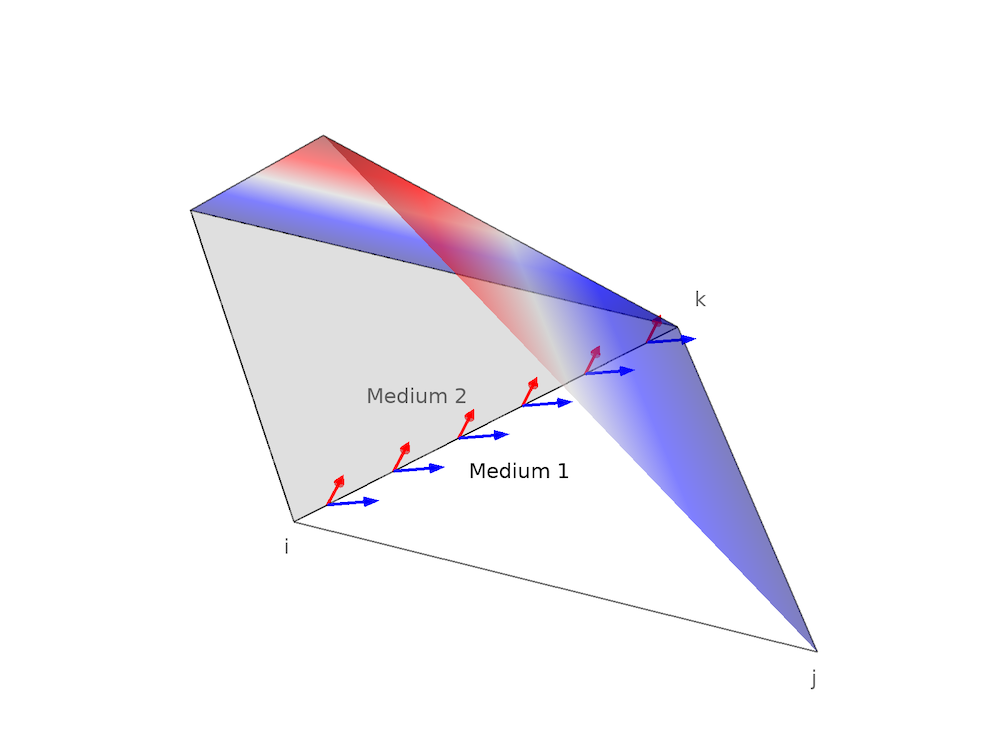Medium 1 and medium 2 share the same boundary, ki. The electric field close to the boundary ki is plotted in blue and red arrows in mediums 1 and 2, respectively. Since the electric potential V is continuous across boundaries, the tangential gradient of V (i.e., the tangential electric field) is continuous. In order words, the boundary condition Eq. (7) is automatically satisfied. Thus, we only need to consider Eq. (6) where surface charges are present. Next, we will show how this could be naturally handled by the FEM.

The derivation of the weak form for Poisson’s equation (10) is not difficult. Multiply the test function \phi to both sides of equation (10) , and the integral over the domain \Omega gives

(14)

\int_{\Omega} \nabla \cdot (\epsilon \nabla \textit{V} )\phi= \int_{\Omega} – \rho \phi

Applying the integration by parts for the left-hand side yields

(15)

-\int_{\Omega} \epsilon \nabla \textit{V} \cdot \nabla \phi + \int_{\partial \Omega} \epsilon \nabla \textit{V} \cdot \textbf{n} \phi= \int_{\Omega} – \rho \phi

with \partial \Omega being the boundary of the domain and \textbf{n} the normal vector oriented away from the domain.

This step is of great importance and has two main advantages:

1. Reducing the maximum order of spatial derivatives, which makes it possible to solve the second-order PDE with linear (first-order) elements
2. Making it clear what the natural boundary condition (automatically satisfied if not consider it) is for the equation

The second integral on the left-hand side disappears if the normal component of \nabla \textit{V} vanishes on the boundary. To clearly see how it works, it is convenient to rewrite equation (15) as

(16)

\int_{\Omega} \textbf{D} \cdot \nabla \phi – \int_{\partial \Omega} \textbf{D} \cdot \textbf{n} \phi= \int_{\Omega} – \rho \phi

where it is clear that the natural boundary condition is \textbf{D} \cdot \textbf{n}=0.

In the Electrostatics interface of the AC/DC Module, this natural boundary condition is set as the default. For situations where the boundary condition is not given by the electric potential but by the electric charge density, it is straightforward to incorporate the boundary condition Eq. (6) to equation (16).

### Curl Elements

It is also possible to use Lagrange-element-based FEM to solve the vector equation (12) by dividing it into different components, \textbf{E}_{x,y,z}. However, it is complicated to implement the boundary conditions, especially for irregular boundaries. Even so, the results could be spurious, since the Lagrange element would enforce each component \textbf{E}_{x,y,z} to be continuous across the boundary, which violates the fact that the normal component of electric field can be discontinuous at material interfaces, especially at boundaries from sharp corners.

Let’s take the Sierpinski Fractal Monopole Antenna model from the Application Library as an example. The surface electric field of the fractal radiator at 1.6 GHz is shown below. It is clear that \textbf{E}_{x,y,z} greatly changes across the edges.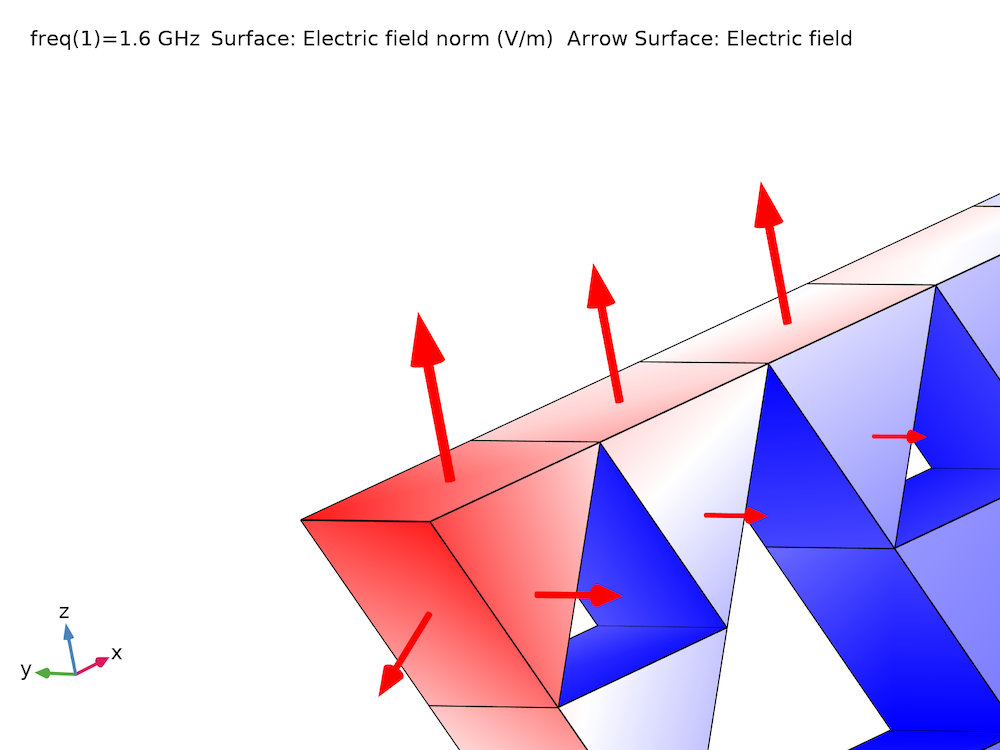As shown earlier, the Lagrange element uses scalar shape functions to approximate the scalar field. It is natural to use vector shape functions to approximate the vector field. For instance, the electric field in equation (12) can be expressed as:

(17)

\textbf{E} = E_i \textbf{W}_i+E_j \textbf{W}_j + E_k \textbf{W}_k

As equation (17) indicates, it is not suitable to add DOFs on nodes, since a vector field also has a direction. To obtain a scalar value, we could add DOFs on each edge, since the vector field component along the edge (tangential component) is a scalar. Then, we rewrite equation (17) as:

(18)

\textbf{E} = E_{ij} \textbf{W}_{ij}+E_{jk} \textbf{W}_{jk} + E_{ki} \textbf{W}_{ki}

Similar to the Lagrange shape function, the tangential component of the vector shape function along one edge is a non-zero constant, and along the other edges, equal to zero. One type of shape function that satisfies the above property is the Whitney 1-form basis function (Ref. 1, Ref. 2), which is expressed as:

(19)

\textbf{W}_{ij} = N_i \nabla N_j – N_j \nabla N_i
\textbf{W}_{jk} = N_j \nabla N_k – N_k \nabla N_j
\textbf{W}_{ki} = N_k \nabla N_i – N_i \nabla N_k

The shape functions of the first-order triangular edge element are plotted below.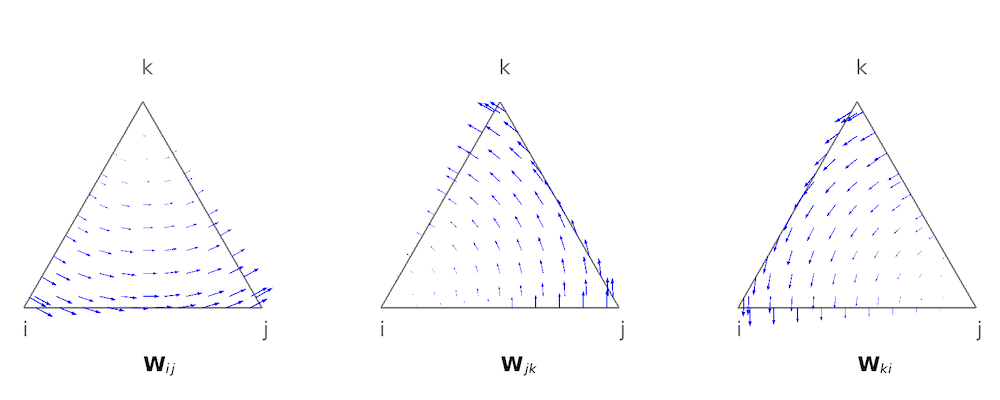From the mathematics point of view, the edge element is conforming in the H(curl) space (Ref. 3), thus it is also called a curl element. In COMSOL Multiphysics, we use the more general name ‘curl element’ since the higher-order elements add more DOFs not only on the edges, but also inside the element. Similar to what we have plotted for two adjacent Lagrange elements, the basis functions of two curl elements sharing the same boundary ki are shown below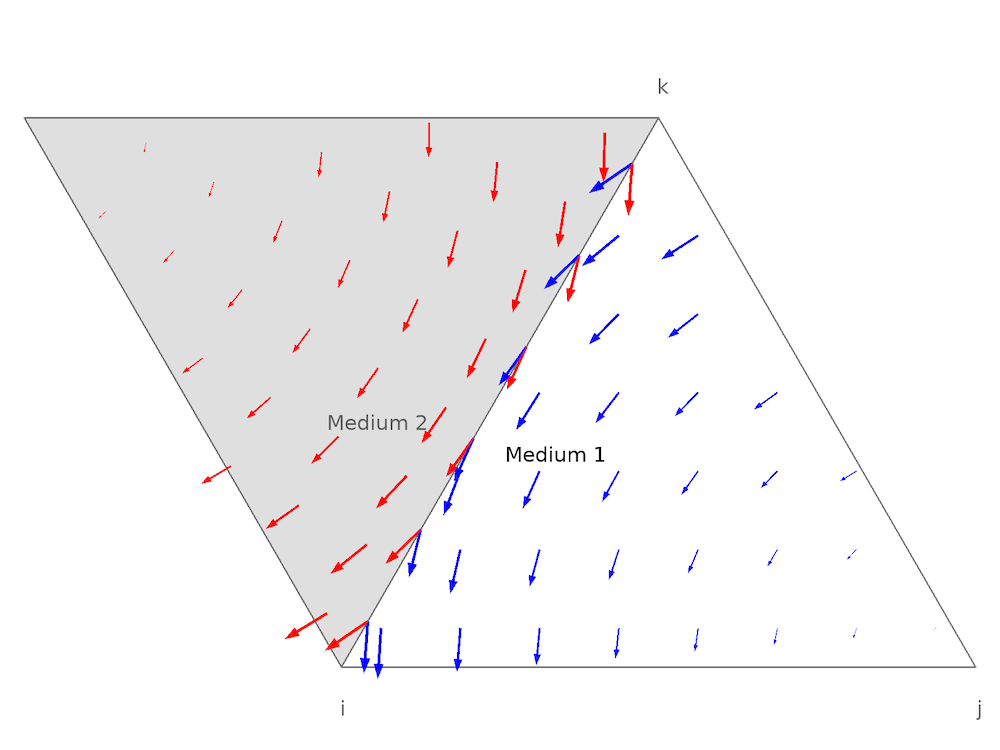We can see that the tangential electric field across the boundary is continuous, which is very similar to the case of using Lagrange elements to solve Poisson equation. Therefore, by using the curl elements, the boundary condition Eq. (7) is automatically fulfilled. Let us then show how boundary Eq. (9) is handled by FEM.

Similar to solving Poisson’s equation, multiply the test function \textbf{W} to both sides of equation (12), and the integral over the domain \Omega gives

(20)

\int_{ \Omega} \nabla \times (\frac {1}{\mu} \nabla \times \textbf{E} ) \textbf{W}=\int_{ \Omega} (\omega^2 \epsilon \textbf{E} – j \omega \textbf{J}) \textbf{W}

Applying the integration by parts for the left-hand side yields

(21)

\int_{ \Omega} (\frac {1}{\mu} \nabla \times \textbf{E} ) (\nabla \times \textbf{W}) + \int_{\partial \Omega} \textbf{n} \times (\frac {1}{\mu} \nabla \times \textbf{E} )\textbf{W} =\int_{ \Omega} (\omega^2 \epsilon \textbf{E} – j \omega \textbf{J}) \textbf{W}

Rewriting the second part of the left-hand side yields

(22)

\int_{ \Omega} (\frac {1}{\mu} \nabla \times \textbf{E} ) (\nabla \times \textbf{W}) -j \omega \int_{\partial \Omega} ( \textbf{n} \times \textbf{H} )\textbf{W} =\int_{ \Omega} (\omega^2 \epsilon \textbf{E} – j \omega \textbf{J}) \textbf{W}

where we can see that the boundary condition Eq. (9) could be easily incorporated into the weak expression.

We have shown that by using the curl elements, the boundary conditions of Maxwell’s equations can be handled naturally. The curl elements enforce the tangential electric field to be continuous and allow the normal electric field to jump across the boundaries. Moreover, it also makes it easier to constrain other boundary conditions (Ref. 4).

For example, in the Electromagnetic Waves, Frequency Domain interface, the Perfect Electric Conductor boundary feature is set as the default to model electrically conducting surfaces, such as metals. The boundary condition enforces the tangential electric field to be zero; i.e., \textbf{n} \times \textbf{E} = \textbf{0}. In the Magnetic Fields interface, the default boundary condition is Magnetic Insulation, which constrains the tangential magnetic vector potential to be zero; i.e., \textbf{n} \times \textbf{A} = \textbf{0}. These boundary conditions could be easily considered by the FEM using pointwise constraints since the unknowns are exactly the tangential fields on the boundary. There are other advantages of using curl elements, such as eliminating spurious solutions, especially for solving electromagnetic wave problems (Ref. 5). However, the natural handling of the boundary conditions should be predominant.

There are a few disadvantages of using curl elements. For example, the linear curl element has local approximation errors of the order O(h), where h is the element size, while using linear Lagrange elements, the local errors converge at O(h^2) (Ref. 6). This is because the curl element is a mixed element where the order of the shape function varies in different directions. For instance, the tangential components of shape function \textbf{W}_{ij} along edge ij and any direction parallel to edge ij are constants, although it is a linear function in other directions. The mixed property can also be shown by looking at the component, for example,

(23)

\textbf{W}_{ij} = N_i \nabla N_j – N_j \nabla N_i
=(a_i+b_i x+c_i y)(b_j, c_j)-(a_j+b_j x+c_j y)(b_i,c_i)
=(d_i + d_{ij} y, d_j – d_{ij} x)

where a,b,c,d are constants depending on the coordinates of the element only.

The x component of \textbf{W}_{ij} is constant along the x axis and is linear along the y axis. The accuracy of the spatial derivatives of each component would be significantly different. For this reason, when postprocessing curl elements, the higher-order spatial derivatives of the fields are not available. Equation (23) also shows that the shape function of the linear curl element can be constant along a specific direction. This makes the local error converge slower than that using a linear Lagrange element. On one hand, this disadvantage can be eliminated by using higher-order curl elements or finer meshes. On the other hand, compared to the advantages of handling boundary conditions naturally, the disadvantages become much less important, since the difficulties encountered by using Lagrange elements to solve equation (12) cannot be compensated by using higher-order shape functions or refining the mesh.

### Summary

In this blog post, starting with Maxwell’s equations and their boundary conditions, we have introduced two basic types of equations that often appear in electromagnetics modeling; i.e., the scalar Poisson’s equation and the vector equation with \nabla \times (\nabla \times .) operator. We have shown that by using the classical Lagrange element to solve Poisson’s equation, the condition of continuous tangential electric fields is satisfied naturally. However, it is not suitable to use the Lagrange element to solve the vector equation due to the difficulties in handling the associated boundary conditions.

We then introduced the curl element, which could satisfy the condition of continuous tangential electric fields naturally. At the same time, we also have shown, by deriving the weak expressions in detail, how other boundary conditions are incorporated in FEM. As last, we talked about some disadvantages of using curl elements, which are much less important than the advantages.

### References

1. H. Whitney, Geometric integration theory, Princeton UP, Princeton, 1957.
2. A. Nentchev, Numerical analysis and simulation in microelectronics by vector finite elements, 2008.
3. J.C. Nédélec, Mixed finite elements in ℝ3 . Numerische Mathematik, 35(3), pp. 315–341, 1980.
4. J.P. Webb, Edge elements and what they can do for you. IEEE Transactions on Magnetics, 29(2), pp.1460–1465, 1993.
5. J.M. Jin, Theory and computation of electromagnetic fields. John Wiley & Sons, 2011.
6. G. Mur, Edge elements, their advantages and their disadvantages. IEEE transactions on magnetics, 30(5), pp.3552–3557, 1994.

#### Categories##### Luiz Fernando de Oliveira
December 30, 2019

Very interesting post!

I just suggest changing the following part:
“D and B are the electric and magnetic flux field intensity” to “flux density”##### Lipeng Liu
December 30, 2019 COMSOL Employee

Hi, Luiz,

Thanks for your comment and suggestion. I have changed ‘flux field intensity’ to ‘flux density’, which is more commonly used.

Lipeng##### Yufan Yan
January 15, 2020

Thank you for the blog! It’s very interesting.

We actually built a few models with curl elements for EMF simulations before in older version. But in the new versions (5.0 and later?), the ‘curl element’ option in the “Discretization”->”Shape Function Type” list is gone. So how could I implement General Form PDE models with curl elements?

(So far we just run them with older version COMSOL, lol.)##### Lipeng Liu
January 15, 2020 COMSOL Employee

Hi Yufan,

The support for ‘curl element’ is always there. Please note that you have to change the ‘Number of dependent variables’ to 2 and 3, for 2D and 3D, respectively, to make it available in the ‘Shape function type’.

Regards,
Lipeng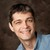##### Josh Thomas
February 20, 2020

Is it possible to apply curl elements to edges in a 3D simulation? I tried using the Pointwise constraint on an edge but I do not see the option in the Shape function type menu. In previous versions, there was a PEC edge option but I don’t see it anymore.##### Lipeng Liu
February 21, 2020 COMSOL Employee

Hi Josh, it is a good question!

Currently, we do not support the curl element discretization to ‘Pointwise Constraint’ on edges in 3D. If you want to do that, use the Lagrange shape function. It works almost in the same way since ‘Shape function type’ in the ‘Pointwise Constraint’ Settings is only used to discretize the constraints. In the end, it will be converted to eliminate the unknowns which are discretized by the ‘Shape function type’ defined in the Physics Interface (Discretization), in your case, the curl element.

However, adding edge ‘Pointwise Constraint’ to a 3D model is generally not recommended. The solution is usually mesh dependent (not converge when refining the mesh) and it might be unphysical. For this reason, the PEC edge constraint option was removed. If you really want to add such constraint, it is suggested to perform a mesh convergence study afterwards since the solution around the edge might be wrong.

Regards,
Lipeng##### Xin Ran Song
August 20, 2020

Hello! I am trying to model the magnetostatics situation (basically equation (11)) using the PDE interface, and realized that there is no built-in curl operator. Do I have to input the equation using weak form? And if so, how? Thank you!##### Lipeng Liu
August 20, 2020 COMSOL Employee

Hi Xinran,

You do not need to type the curl operator and it is not recommended to write the expressions. Instead, you can simply use (curlAx, curlAy, curlAz) if your dependent field name is A. In COMSOL, curlAx = x component of curl(A) = Bx. You can find more details about the usage of curl element in COMSOL Reference Manual (Chapter: Elements and Shape Functions).

Regards,
Lipeng

EXPLORE COMSOL BLOG